Theory and Modern Applications

# Existence and uniqueness of solution for a class of nonlinear fractional differential equations

## Abstract

In this paper, we present some new existence and uniqueness results for nonlinear fractional differential equations with a kind of general irregular boundary condition in Banach space by using a fixed-point theorem and contraction mapping principle. Moreover, the boundary condition is extended, therefore, some conclusions from other references are special cases of our results.

MSC:26A33, 34A08.

## 1 Introduction

The fractional derivative (or called the noninteger order derivative) was invented by Leibnitz and L’Hospital around the seventeenth century and the related calculus was developed in about 200 years later . Although the concepts and calculus of fractional differential equations (FDEs) are several centuries old, they are realized only some decades ago that these derivatives can be employed in modeling the real world excellently [4, 5]. Thus in recent years, the FDEs are extensively applied to many fields of the science and engineering, such as heat transfer problem of blast furnace , financial market’s behaviors , polymer physics [8, 9], material molding , electrocircuit , chemical reaction , and biology . Boundary value problems (BVPs) of differential equations could be seen in scientific and engineering disciplines and have been investigated systematically by many authors; for more details, we refer to  and related references therein. More precisely, there has been much work of extending the theory of BVPs from classical differential equations to the sense of fractional differential equations. For instance, in , a kind of irregular boundary value problems is discussed. In , the existence of fractional differential equations with anti-periodic boundary conditions is studied, and in , Z. Bai investigates the positive solutions of nonlinear fractional boundary value problems. For more details of positive solutions of fractional differential systems and fractional BVPs with nonlocal conditions, see [20, 21] and references therein.

In this paper, we mainly study a kind of general irregular boundary value problem for nonlinear fractional equations of fractional order $q\in \left(1,2\right]$. Motivated by , we consider the following irregular boundary value problem

$\left\{\begin{array}{c}{}^{c}D^{q}x\left(t\right)=f\left(t,x\left(t\right)\right),\phantom{\rule{1em}{0ex}}0\le t\le \pi ,1
(1)

where ${}^{c}D^{q}$ is the Caputo fractional derivative of order q which is defined in next section and $f:\left[0,\pi \right]×X\to X$. Here, $\left(X,\parallel \cdot \parallel \right)$ is a Banach space and $\mathrm{\Omega }={C}^{1}\left(\left[0,\pi \right],X\right)$ denotes the Banach space of all continuously differentiable functions from $\left[0,\pi \right]$ to X endowed with a topology of uniform convergence with the norm denoted by $\parallel \cdot \parallel$.

## 2 Preliminaries

We give some basic definitions and properties of the fractional calculus theory which are essential in this paper. They are easily found in [2, 6, 24, 25].

Definition 2.1 ()

A real function $f\left(x\right)>0$, $x>0$, is said to be in the space ${C}_{\mu }$, $\mu \in R$ if there exists a real number p (>μ), such that $f\left(x\right)={x}^{p}{f}_{1}\left(x\right)$, where ${f}_{1}\left(x\right)\in C\left[0,+\mathrm{\infty }\right)$, and it is said to be in the space ${C}_{\mu }^{m}$ if and only if ${f}^{\left(m\right)}\in {C}_{\mu }$, $m\in N$.

Definition 2.2 ([2, 6])

The Riemann-Liouville fractional integral operator of order $q\ge 0$, of a function $x\in {C}_{\mu }$, $\mu >-1$, is defined as

${I}^{q}x\left(t\right)=\frac{1}{\mathrm{\Gamma }\left(q\right)}{\int }_{0}^{t}{\left(t-\tau \right)}^{q-1}x\left(\tau \right)\phantom{\rule{0.2em}{0ex}}d\tau ,\phantom{\rule{1em}{0ex}}q>0,t>0.$
(2)

Particularly, if $q=0$, ${I}^{0}x\left(t\right)=x\left(t\right)$.

Definition 2.3 ([2, 6])

The fractional derivative of $x\left(t\right)$ in the Caputo sense is defined as

${}^{c}D^{q}x\left(t\right)=\frac{1}{\mathrm{\Gamma }\left(n-q\right)}{\int }_{0}^{t}{\left(t-\tau \right)}^{n-q-1}{x}^{\left(n\right)}\left(\tau \right)\phantom{\rule{0.2em}{0ex}}d\tau ,$

for $n-1, $n\in {N}^{+}$, $t>0$, $x\in {C}_{-1}^{n}$.

Lemma 2.1 ()

For $q>0$, the general solution of the fractional differential equation ${}^{c}D^{q}x\left(t\right)=0$ is given by

$x\left(t\right)={c}_{0}+{c}_{1}t+{c}_{2}{t}^{2}+\cdots +{c}_{n-1}{t}^{n-1},$

where ${c}_{i}\in R$, $i=0,1,2,\dots ,n-1$ ($n=\left[q\right]+1$, $\left[q\right]$ denotes the integer part of the real number q).

Lemma 2.2 ()

For any $x\in {C}_{-1}^{n}$, then

${I}^{q}{\left\{}^{c}{D}^{q}x\left(t\right)\right\}=x\left(t\right)+{c}_{0}+{c}_{1}t+{c}_{2}{t}^{2}+\cdots +{c}_{n-1}{t}^{n-1},$
(3)

where ${c}_{i}\in R$, $i=1,2,\dots ,n-1$ ($n=\left[q\right]+1$). Here, ${I}^{q}$ denotes the Riemann-Liouville fractional integral operator of order q.

Lemma 2.3 For any given $\sigma \in {C}^{1}\left[0,\pi \right]$, the unique solution of the boundary value problem

$\left\{\begin{array}{c}{}^{c}D^{q}x\left(t\right)=\sigma \left(t\right),\phantom{\rule{1em}{0ex}}0\le t\le \pi ,1
(4)

is given by

$x\left(t\right)={\int }_{0}^{t}\frac{{\left(t-s\right)}^{q-1}}{\mathrm{\Gamma }\left(q\right)}\sigma \left(s\right)\phantom{\rule{0.2em}{0ex}}ds-\frac{{M}_{3}}{{M}_{1}}-\frac{{M}_{2}}{{M}_{1}}t,\phantom{\rule{1em}{0ex}}{M}_{1}\ne 0,$
(5)

whereProof According to Eq. (3), the general solution of Eq. (4) can be written as

$x\left(t\right)={I}^{q}\sigma \left(t\right)-{c}_{0}-{c}_{1}t={\int }_{0}^{t}\frac{{\left(t-s\right)}^{q-1}}{\mathrm{\Gamma }\left(q\right)}\sigma \left(s\right)\phantom{\rule{0.2em}{0ex}}ds-{c}_{0}-{c}_{1}t,$
(6)

where ${c}_{0}$, ${c}_{1}$ are arbitrary constants. Then differentiating Eq. (6) to t yields that

${x}^{\prime }\left(t\right)={\int }_{0}^{t}\frac{{\left(t-s\right)}^{q-2}}{\mathrm{\Gamma }\left(q-1\right)}\sigma \left(s\right)\phantom{\rule{0.2em}{0ex}}ds-{c}_{1}.$
(7)

Using the boundary conditions in Eq. (4), we have the following equations from Eq. (6) and Eq. (7):

$\left\{\begin{array}{c}{\left(-1\right)}^{{\alpha }_{1}}\left(-{c}_{1}\right)+{\left(-1\right)}^{{\beta }_{1}}\left({\int }_{0}^{\pi }\frac{{\left(\pi -s\right)}^{q-2}}{\mathrm{\Gamma }\left(q-1\right)}\sigma \left(s\right)\phantom{\rule{0.2em}{0ex}}ds-{c}_{1}\right)\hfill \\ \phantom{\rule{1em}{0ex}}+a\left(-{c}_{0}\right)+b\left({\int }_{0}^{\pi }\frac{{\left(\pi -s\right)}^{q-1}}{\mathrm{\Gamma }\left(q\right)}\sigma \left(s\right)\phantom{\rule{0.2em}{0ex}}ds-{c}_{0}-{c}_{1}\pi \right)=0,\hfill \\ {\left(-1\right)}^{{\alpha }_{2}}\left(-{c}_{0}\right)+{\left(-1\right)}^{{\beta }_{2}}\left({\int }_{0}^{\pi }\frac{{\left(\pi -s\right)}^{q-1}}{\mathrm{\Gamma }\left(q\right)}\sigma \left(s\right)\phantom{\rule{0.2em}{0ex}}ds-{c}_{0}-{c}_{1}\pi \right)=0.\hfill \end{array}$
(8)

It follows that

$\left\{\begin{array}{c}{c}_{0}=\frac{{A}_{0}{B}_{2}-{B}_{0}{B}_{1}}{{A}_{1}{B}_{2}-{A}_{2}{B}_{1}},\hfill \\ {c}_{1}=\frac{{A}_{1}{B}_{0}-{A}_{0}{A}_{2}}{{A}_{1}{B}_{2}-{A}_{2}{B}_{1}},\hfill \end{array}$
(9)

where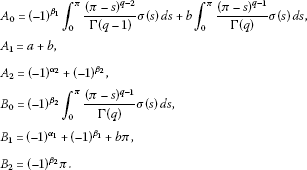Setting ${M}_{1}={A}_{1}{B}_{2}-{A}_{2}{B}_{1}$, ${M}_{2}={A}_{1}{B}_{0}-{A}_{0}{A}_{2}$, ${M}_{3}={A}_{0}{B}_{2}-{B}_{0}{B}_{1}$, we get Eq. (5). This completes the proof. □

The boundary value condition in BVP (1) is more general. If $a=b=0$, and ${\alpha }_{1}={\alpha }_{2}={\beta }_{1}={\beta }_{2}=0$, the boundary value condition becomes antiperiodic boundary value condition. If $a=b=0$, ${\alpha }_{1}={\alpha }_{2}=0$, and ${\beta }_{1}={\beta }_{2}=1$ (or $a=b=0$, ${\alpha }_{1}={\alpha }_{2}=1$, and ${\beta }_{1}={\beta }_{2}=0$), the boundary value condition becomes periodic boundary value condition. Therefore, the above two kinds of boundary value conditions are spacial cases of the irregular boundary value condition considered in BVP (1). Furthermore, it is easy to conclude that BVP (1) is solvable with antiperiodic boundary value condition and unsolvable with periodic boundary condition.

Corollary 1 A unique solution of a general linear second order irregular boundary value problem can be obtained by fixing $q=2$ in Lemma 2.3, which is a meaningful result. Moreover, the condition ${M}_{1}\ne 0$ is necessary to guarantee the existence of solution (see Eq. (5)).

Remark 2.1 In some references (such as [14, 26]), the σ is always restricted in $C\left[0,\pi \right]$, i.e., a continuous function. But in our opinion, this restriction would arise some little problems. For ensuring that $x\left(t\right)$ in Eq. (5) makes sense, σ has to be at least first order continuously differentiable, i.e., $\sigma \in {C}^{1}\left[0,\pi \right]$. Otherwise, the Lemma 2.3 is not true since the second order derivative $\frac{{d}^{2}}{d{t}^{2}}x\left(t\right)$ does not exist when $q=2$.

The following theorem is needed to prove the existence of at least one solution of the general irregular boundary value problem, and its detailed proof can be seen in [23, 27, 28].

Theorem 2.1 (Krasnoselskii, , Theorem 4.4.1])

Let M be a closed convex and nonempty subset of a Banach space X. Suppose that Φ and Ψ map M into X and that

1. (i)

$\mathrm{\Phi }x+\mathrm{\Psi }y\in M$ ($\mathrm{\forall }x,y\in M$),

2. (ii)

Φ is compact and continuous,

3. (iii)

Ψ is a contraction mapping.

Then there exists ${y}^{\ast }$ in M such that

$\mathrm{\Phi }{y}^{\ast }+\mathrm{\Psi }{y}^{\ast }={y}^{\ast }.$

## 3 Main results

In this section, we propose our main results of existence and uniqueness of general irregular boundary value problem. Firstly, we prove an existence and uniqueness theorem based on the contraction mapping principle in Banach space [26, 28].

Theorem 3.1 Let $f:\left[0,\pi \right]×X\to X$ be a first-order continuously differentiable function satisfying the condition

$\parallel f\left(t,x\right)-f\left(t,y\right)\parallel \le L\parallel x-y\parallel ,\phantom{\rule{1em}{0ex}}\mathrm{\forall }t\in \left[0,\pi \right],x,y\in X.$

Then the boundary value problem (1) has a unique solution provided $\mathrm{\Delta }<1$, where

$\begin{array}{rcl}\mathrm{\Delta }& =& \frac{L}{|{M}_{1}|\mathrm{\Gamma }\left(q+1\right)}\left\{|{M}_{1}|{\pi }^{q}+{\pi }^{q}|{\left(-1\right)}^{{\alpha }_{1}+{\beta }_{2}}+\left(2q+1\right){\left(-1\right)}^{{\beta }_{1}+{\beta }_{2}}\\ +q{\left(-1\right)}^{{\alpha }_{2}+{\beta }_{1}}+\pi \left(a{\left(-1\right)}^{{\beta }_{2}}-b{\left(-1\right)}^{{\alpha }_{2}}\right)|\right\},\end{array}$

and ${M}_{1}$ is defined in Lemma 2.3.

Proof Now we define $F:\mathrm{\Omega }\to \mathrm{\Omega }$ by

$\begin{array}{rcl}\left(Fx\right)\left(t\right)& =& {\int }_{0}^{t}\frac{{\left(t-s\right)}^{q-1}}{\mathrm{\Gamma }\left(q\right)}f\left(s,x\left(s\right)\right)\phantom{\rule{0.2em}{0ex}}ds-\frac{{\left(-1\right)}^{{\beta }_{1}+{\beta }_{2}}\pi }{{M}_{1}}{\int }_{0}^{\pi }\frac{{\left(\pi -s\right)}^{q-2}}{\mathrm{\Gamma }\left(q-1\right)}f\left(s,x\left(s\right)\right)\phantom{\rule{0.2em}{0ex}}ds\\ +\frac{\left({\left(-1\right)}^{{\alpha }_{1}+{\beta }_{2}}+{\left(-1\right)}^{{\beta }_{1}+{\beta }_{2}}\right)}{{M}_{1}}{\int }_{0}^{\pi }\frac{{\left(\pi -s\right)}^{q-1}}{\mathrm{\Gamma }\left(q\right)}f\left(s,x\left(s\right)\right)\phantom{\rule{0.2em}{0ex}}ds\\ -\frac{t\left(a{\left(-1\right)}^{{\beta }_{2}}-b{\left(-1\right)}^{{\alpha }_{2}}\right)}{{M}_{1}}{\int }_{0}^{\pi }\frac{{\left(\pi -s\right)}^{q-1}}{\mathrm{\Gamma }\left(q\right)}f\left(s,x\left(s\right)\right)\phantom{\rule{0.2em}{0ex}}ds\\ +\frac{t\left({\left(-1\right)}^{{\alpha }_{2}+{\beta }_{1}}+{\left(-1\right)}^{{\beta }_{1}+{\beta }_{2}}\right)}{{M}_{1}}{\int }_{0}^{\pi }\frac{{\left(\pi -s\right)}^{q-2}}{\mathrm{\Gamma }\left(q-1\right)}f\left(s,x\left(s\right)\right)\phantom{\rule{0.2em}{0ex}}ds,\end{array}$

and let us define ${M}_{0}={sup}_{t\in \left[0,\pi \right]}\parallel f\left(t,0\right)\parallel$ and

$\begin{array}{rcl}r& \ge & \frac{{M}_{0}}{\left(1-\mu \right)|{M}_{1}|\mathrm{\Gamma }\left(q+1\right)}\left\{|{M}_{1}|{\pi }^{q}+{\pi }^{q}|{\left(-1\right)}^{{\alpha }_{1}+{\beta }_{2}}+\left(2q+1\right){\left(-1\right)}^{{\beta }_{1}+{\beta }_{2}}\\ +q{\left(-1\right)}^{{\alpha }_{2}+{\beta }_{1}}+\pi \left(a{\left(-1\right)}^{{\beta }_{2}}-b{\left(-1\right)}^{{\alpha }_{2}}\right)|\right\},\end{array}$

where μ is a real number satisfying $\mathrm{\Delta }\le \mu <1$, and Ω is well defined in Section 1. As the similar idea in , we denote ${B}_{r}=\left\{x\in \mathrm{\Omega }:\parallel x\parallel \le r\right\}$. Furthermore, for any $x\in {B}_{r}$, we have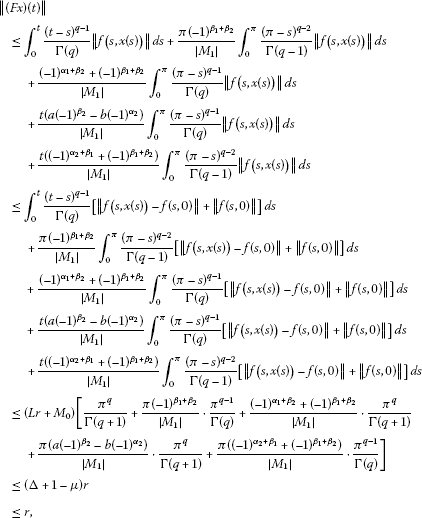thus $F\left({B}_{r}\right)\subset {B}_{r}$ is verified.

Now for any $x,y\in \mathrm{\Omega }$ and for each $t\in \left[0,\pi \right]$, we obtain thatas $\mathrm{\Delta }\le 1$, therefore, F is a contraction. By using the conclusion of contraction mapping principle in Banach space, we complete our proof. □

Remark 3.1 We restrict the function $f\left(t,\cdot \right)$ to be in ${L}^{1}$ at least, which is different from the hypothetical conditions of $f\left(t,x\right)$ in . For the simplicity of our proof in Theorem 3.1, $f\left(t,x\right)$ is a first-order continuously differentiable function satisfying Lipschitz condition.

Theorem 3.2 Assume that $f:\left[0,\pi \right]×X\to X$ is a first-order continuously differentiable function and maps bounded subsets of $\left[0,\pi \right]×X$ into relative compact subsets of X, satisfying the following assumptions:

(A1) $\parallel f\left(t,x\right)-f\left(t,y\right)\parallel \le L\parallel x-y\parallel$, $\mathrm{\forall }t\in \left[0,\pi \right]$, $x,y\in X$,

(A2) $\parallel f\left(t,x\right)\parallel \le h\left(t\right)$, $\mathrm{\forall }\left(t,x\right)\in \left[0,\pi \right]×X$, and $h\in {L}^{1}\left(\left[0,\pi \right],{R}^{+}\right)$.

If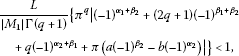then the general irregular boundary value problem (1) has at least one solution on $\left[0,\pi \right]$.

Proof We first restrict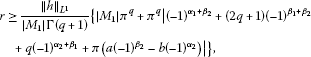and denote ${B}_{r}=\left\{x\in \mathrm{\Omega }:\parallel x\parallel \le r\right\}$. Moreover, the operators Φ and Ψ are defined on ${B}_{r}$ as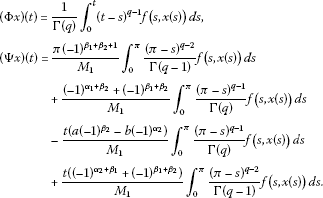We could find that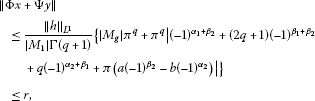for $x,y\in {B}_{r}$. Therefore, we have $\mathrm{\Phi }x+\mathrm{\Psi }y\in {B}_{r}$. According to the assumption (A1) of our theorem, Ψ is a contraction mapping sincewhere ${M}_{1}$ is well defined in Theorem 3.1. By the definition of Φ, we can easily check that Φ is continuous (since $f\left(t,x\right)$ is continuous) and uniformly bounded on ${B}_{r}$ as

$\parallel \mathrm{\Phi }x\parallel \le \frac{{\pi }^{q}{\parallel h\parallel }_{{L}^{1}}}{\mathrm{\Gamma }\left(q+1\right)}.$

Now we prove the compactness of the operator Φ. We denote ${f}_{max}={sup}_{\left(t,x\right)\in \left[0,\pi \right]×{B}_{r}}\parallel f\left(t,x\right)\parallel$, and for any $\left({t}_{1},x\left({t}_{1}\right)\right)$ and $\left({t}_{2},x\left({t}_{2}\right)\right)$ in domain, we have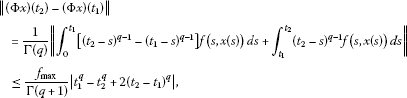which is independent of x. Thus, Φ is equicontinuous. According to the fact that Φ maps bounded subsets into relatively compact subsets, we know that $\mathrm{\Phi }\left(S\right)\left(t\right)$ is relatively compact in X for every t, where S is a bounded subset of Ω. So $\mathrm{\Phi }\left(\cdot \right)$ is relatively compact on ${B}_{r}$. Thus, by using the Arzela-Ascoli theorem from functional analysis, Φ is compact on ${B}_{r}$. Finally, we have the conclusion that the general irregular boundary value problem (1) has at least one solution on $\left[0,\pi \right]$ since all assumptions of Theorem 2.1 are satisfied, so the proof is completed. □

Furthermore, we have the next remark about the Green’s function of boundary value problem (1).

Remark 3.2 The Green’s function $G\left(t,s\right)$ of boundary value problem (1) could be written as

$G\left(t,s\right)=\left\{\begin{array}{c}\frac{{\left(\pi -s\right)}^{q-2}}{{M}_{1}\mathrm{\Gamma }\left(q-1\right)}\left\{t\left({\left(-1\right)}^{{\alpha }_{2}+{\beta }_{1}}+{\left(-1\right)}^{{\beta }_{1}+{\beta }_{2}}\right)-\pi {\left(-1\right)}^{{\beta }_{1}+{\beta }_{2}}\right\}\hfill \\ \phantom{\rule{1em}{0ex}}+\frac{{\left(\pi -s\right)}^{q-1}}{{M}_{1}\mathrm{\Gamma }\left(q\right)}\left\{\left({\left(-1\right)}^{{\alpha }_{1}+{\beta }_{2}}+{\left(-1\right)}^{{\beta }_{1}+{\beta }_{2}}\right)-t\left(a{\left(-1\right)}^{{\beta }_{1}}-b{\left(-1\right)}^{{\alpha }_{2}}\right)\right\},\hfill \\ \phantom{\rule{1em}{0ex}}0\le t
(10)

Hence, by taking different $q\in \left(1,2\right]$, we obtain their Green’s function as Eq. (10). Expressly, if $q=2$, a Green’s function of second order general irregular boundary problem is shown, see  and the last reference it cites. There is another truth, that is, the conclusion of  is a special case by fixing ${\alpha }_{i}=0$, $i=1,2$, and ${\beta }_{1}=\theta$, ${\beta }_{2}=\theta +1$, $a=0$ of this paper.

## 4 Two examples

Example 4.1 Consider the following boundary value problem:

$\left\{\begin{array}{c}{}^{c}D^{1.88}x\left(t\right)=\frac{1}{{e}^{t}+36}\cdot \frac{|x|}{1+|x|},\phantom{\rule{1em}{0ex}}t\in \left[0,\pi \right],\hfill \\ {x}^{\prime }\left(0\right)-{x}^{\prime }\left(\pi \right)-2x\left(0\right)+x\left(\pi \right)=0,\hfill \\ x\left(0\right)+x\left(\pi \right)=0.\hfill \end{array}$
(11)

Here, $f\left(t,x\right)=\frac{1}{{e}^{t}+36}\cdot \frac{|x|}{1+|x|}$, $q=1.88$, $a=-2$, $b=1$. As $\parallel f\left(t,x\right)-f\left(t,y\right)\parallel \le \frac{1}{36}\parallel x-y\parallel$, therefore, the assumption in Theorem 2.1 is tenable with $L=\frac{1}{36}$. Moreover,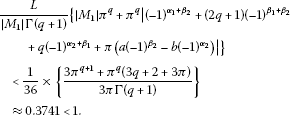Thus, according to Theorem 3.1, the boundary value problem (11) has a unique solution on $\left[0,\pi \right]$.

Example 4.2 Consider the following boundary value problem:

$\left\{\begin{array}{c}{}^{c}D^{1.3}x\left(t\right)=\frac{1}{{e}^{t}+365}\cdot \frac{|x|}{1+|x|},\phantom{\rule{1em}{0ex}}t\in \left[0,\pi \right],\hfill \\ {x}^{\prime }\left(0\right)-{x}^{\prime }\left(\pi \right)+0.55x\left(0\right)+3x\left(\pi \right)=0,\hfill \\ x\left(0\right)+x\left(\pi \right)=0.\hfill \end{array}$
(12)

Here, $f\left(t,x\right)=\frac{1}{{e}^{t}+365}\cdot \frac{|x|}{1+|x|}$, $q=1.3$, $a=0.55$, $b=3$. As $\parallel f\left(t,x\right)-f\left(t,y\right)\parallel \le \frac{1}{365}\parallel x-y\parallel$, therefore, the assumption (A1) in Theorem 3.2 is tenable with $L=\frac{1}{365}$. Moreover,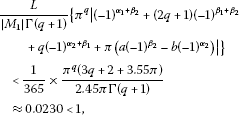and $\parallel f\left(t,x\right)\parallel \le \frac{1}{{e}^{t}+365}=h\left(t\right)$ satisfies the assumption condition (A2) in Theorem 3.2. Thus, according to Theorem 3.2, the boundary value problem (12) has at least one solution on $\left[0,\pi \right]$.

## 5 Conclusions

In this paper, we mainly discuss the existence of solutions for a kind of irregular boundary value problem of nonlinear fractional differential equations. Unlike the restrictions in some references, we give a different opinion about the continuity and differentiation of derivative in the discussed boundary value problem. There are two important points to make here. Firstly, the different restriction of derivative we give is necessary to guarantee the existence of the second-order derivative. Generally speaking, taking BVP (1) as an example, the function $f\left(t,x\left(t\right)\right)$ is necessary to have $\left[q\right]$th order derivative to guarantee the existence of solution could be $\left(\left[q\right]+1\right)$th order differentiable. Secondly, we show a kind of more general boundary condition than that in some references, and some conclusions from other references are special cases of our results.

Finally, the recent applications of differential equations with fractional order as models in more and more fields of science and engineering makes it necessary to study the qualitative theory of such equations, and we hope that our work could make some contributions in this direction.

## References

1. Kilbas AA, Srivasfava HM, Trujillo JJ: Theory and Applications of Fractional Differential Equations. Elsevier, Amsterdam; 2006.

2. Diethelm K: The Analysis of Fractional Differential Equations. Springer, Berlin; 2010.

3. Oldham KB, Spanier J: The Fractional Calculus: Theory and Applications of Differentiation and Integration to Arbitrary Order. Academic Press, San Diego; 1974.

4. Sabatier J, Agrawal OP, Machado JAT (Eds): Advances in Fractional Calculus: Theoretical Developments and Applications in Physics and Engineering. Springer, Dordrecht; 2007.

5. Kilbas AA, Trujillo JJ: Differential equations of fractional order: methods, results and problem. Appl. Anal. 2001, 78: 153–192. 10.1080/00036810108840931

6. Podlubny I: Fractional Differential Equations. Academic Press, San Diego; 1999.

7. Chen WC: Nonlinear dynamics and chaos in a fractional-order financial system. Chaos Solitons Fractals 2008, 36: 1305–1314. 10.1016/j.chaos.2006.07.051

8. Friedrich C: Mechanical stress relaxation in polymers: fractional integral model versus fractional differential model. J. Non-Newton. Fluid Mech. 1993, 46: 307–314. 10.1016/0377-0257(93)85052-C

9. Müller S, Kästner M, Ulbricht JBV: A nonlinear fractional viscoelastic material model for polymers. Comput. Mater. Sci. 2011, 50: 2938–2949. 10.1016/j.commatsci.2011.05.011

10. Pritz T: Five-parameter fractional derivative model for polymeric damping materials. J. Sound Vib. 2003, 265: 935–952. 10.1016/S0022-460X(02)01530-4

11. Sadli I, Urbain M, Hinaje M, Martin JP, Raël S, Davat B: Contributions of fractional differentiation to the modelling of electric double layer capacitance. Energy Convers. Manag. 2010, 51: 2993–2999. 10.1016/j.enconman.2010.06.045

12. Gupalo IP, Novikov VA, Riazantsev IS: Continuous-flow system with fractional order chemical reaction in the presence of axial dispersion. J. Appl. Math. Mech. 1981, 45: 213–216. 10.1016/0021-8928(81)90037-X

13. Magin RL: Fractional calculus models of complex dynamics in biological tissues. Comput. Math. Appl. 2010, 59: 1586–1593. 10.1016/j.camwa.2009.08.039

14. Ahmad B: Existence of solutions for irregular boundary value problems of nonlinear fractional differential equations. Appl. Math. Lett. 2010, 23: 390–394. 10.1016/j.aml.2009.11.004

15. Ahmad B:Existence of solutions for fractional differential equations of order $q\in \left(2,3\right]$ with anti-periodic boundary conditions. J. Appl. Math. Comput. 2010, 34: 385–391. 10.1007/s12190-009-0328-4

16. Muslim M: Existence and approximation of solutions to fractional differential equations. Math. Comput. Model. 2009, 49: 1164–1172. 10.1016/j.mcm.2008.07.013

17. Lakshmikantham V: Theory of fractional functional differential equations. Nonlinear Anal. 2008, 69: 3337–3343. 10.1016/j.na.2007.09.025

18. Lakshmikantham V, Vatsala AS: Basic theory of fractional differential equations. Nonlinear Anal. 2008, 69: 2677–2682. 10.1016/j.na.2007.08.042

19. Bai ZB, Lü HS: Positive solutions for boundary value problem of nonlinear fractional differential equation. J. Math. Anal. Appl. 2005, 311: 495–505. 10.1016/j.jmaa.2005.02.052

20. Goodrich CS: Existence and uniqueness of solutions to a fractional difference equation with nonlocal conditions. Comput. Math. Appl. 2011, 61: 191–202. 10.1016/j.camwa.2010.10.041

21. Goodrich CS: Existence of a positive solution to a system of discrete fractional boundary value problems. Appl. Math. Comput. 2011, 217: 4740–4753. 10.1016/j.amc.2010.11.029

22. Babakhani A, Gejji VD: Existence of positive solutions of nonlinear fractional differential equations. J. Math. Anal. Appl. 2003, 278: 434–442. 10.1016/S0022-247X(02)00716-3

23. Bassam M: Some existence theorems on differential equations of generalized order. J. Reine Angew. Math. 1965, 218: 70–78.

24. Momani S, Odibat Z: Numerical approach to differential equations of fractional order. J. Comput. Appl. Math. 2007, 207: 96–110. 10.1016/j.cam.2006.07.015

25. Lakshmikantham V, Leela S, Devi JV: Theory of Fractional Dynamic Systems. Cambridge Academic Publishers, Cambridge; 2009.

26. Zhang SQ: Existence of solution for a boundary value problem of fractional order. Acta Math. Sci. 2006, 26: 220–228.

27. Granas A, Dugundji J: Fixed Point Theory. Springer, New York; 2003.

28. Smart DR: Fixed Point Theorems. Cambridge University Press, Cambridge; 1980.

## Acknowledgements

We would like to thank the main editor and anonymous referees for their valuable comments and suggestions leading to improvement of this paper. This paper was supported by the Graduate degree thesis Innovation Foundation of Central South University (No. 2010ssxt119). The second author was supported by the National Foundation for Studying Abroad (No. 201206370079).

## Author information

Authors

### Corresponding author

Correspondence to Yufeng Xu.

### Competing interests

The authors declare that they have no competing interests.

### Authors’ contributions

The authors have made the same contribution. All authors read and approved the final manuscript.

## Rights and permissions

Reprints and Permissions

Ma, S., Xu, Y. & Yue, W. Existence and uniqueness of solution for a class of nonlinear fractional differential equations. Adv Differ Equ 2012, 133 (2012). https://doi.org/10.1186/1687-1847-2012-133

• Accepted:

• Published:

• DOI: https://doi.org/10.1186/1687-1847-2012-133

### Keywords

• nonlinear fractional differential equations
• general irregular boundary conditions
• existence
• fixed-point theorem